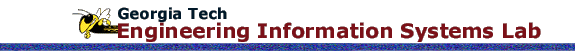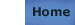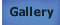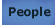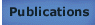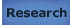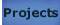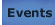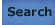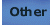# Parallel Methods For High-Performance Finite Element Methods Based On Sparsity

## Reference

Chuang, Shih-Chang (1993) Doctoral Thesis, Georgia Institute of Technology, Atlanta.

## Abstract

Finite element methods are the basis for numerical solutions to mechanical engineering problems. Sparse matrices are an integral part of finite element analysis, and the solution to the associated simultaneous equations can be 75 percent of the total computation time. Realistic engineering problems using such analysis typically require excessively large computation time. Parallel supercomputers have the potential for significantly increasing calculation speeds in order to meet these computational requirements. Yet achieving these increased computation speeds requires consideration of many issues such as numerical methods, parallel strategies, information storage, mapping schemes, etc. Only a few studies exist based on use of sparse matrix methods to improve performance, and none have explored the benefit of sparse parallel algorithms for finite element computations. This study deals specifically with the design, implementation and application of parallel sparse matrix methods for finite element solutions. Areas of research include extensions to the Jacobi, Choleski matrix decomposition, and conjugate-gradient methods. An innovative method is proposed wherein the sparsity of a matrix can be used to reduce computation time and storage. The strategies of sparse matrix algorithms are exploited in relation to the development of new parallel supercomputer architecture associated with high-performance computing. Design methods for dealing with the impact of appropriate data management and mapping strategies have been addressed. In particular, the results consider how such strategies can be linked within the structure of a finite element system and have been tested in existing finite element systems.

Manuscript: order via UMI